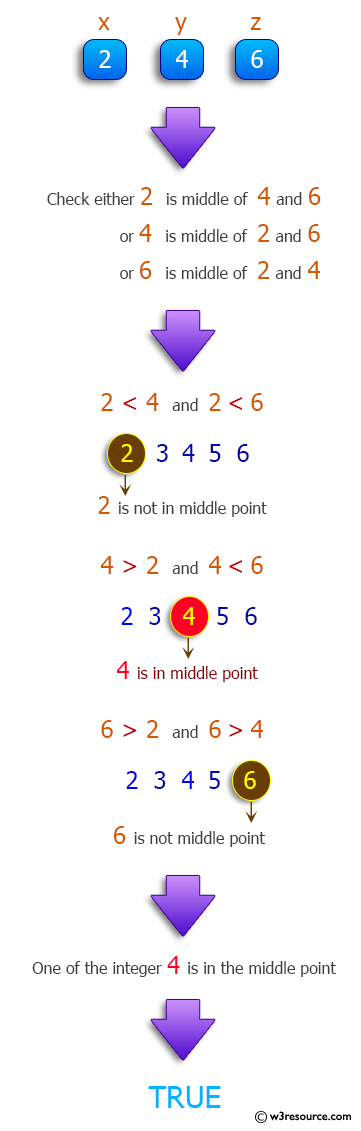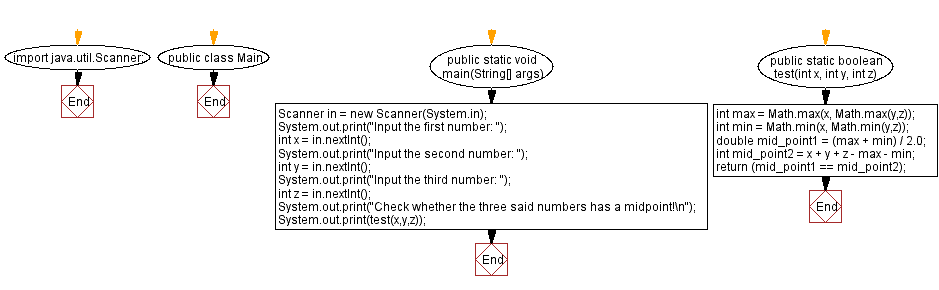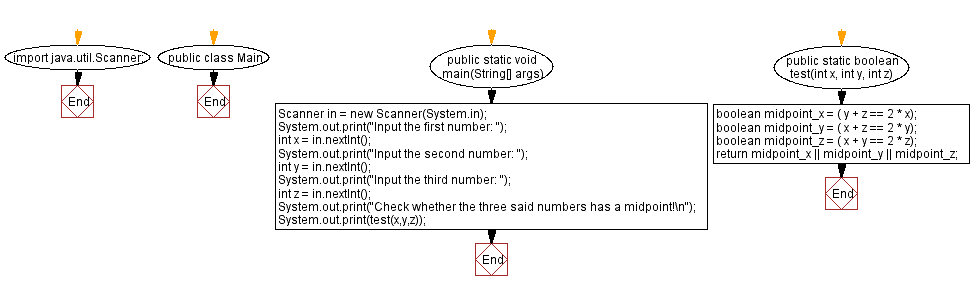﻿ Java: Accept three integers and return the middle one# Java Method Exercises: Accept three integers and return the middle one

## Java Method: Exercise-19 with Solution

Write a Java method that accepts three integers and returns true if one of them is the middle point between the other two integers, otherwise false.

Pictorial Presentation:Sample data:
(2, 4, 6) -> true
(2, 8, 5) -> true
(6, 6, 6) -> true
(20, 7, -6) -> true
(1, 2, 5) -> false
(5, 1, 5) -> false
(3, 9, 14) -> false

Sample Solution-1:

Java Code:

``````import java.util.Scanner;
public class Main {
public static void main(String[] args)
{
Scanner in = new Scanner(System.in);
System.out.print("Input the first number: ");
int x = in.nextInt();
System.out.print("Input the second number: ");
int y = in.nextInt();
System.out.print("Input the third number: ");
int z = in.nextInt();
System.out.print("Check whether the three said numbers has a midpoint!\n");
System.out.print(test(x,y,z));
}

public static boolean test(int x, int y, int z){
int max = Math.max(x, Math.max(y,z));
int min = Math.min(x, Math.min(y,z));
double mid_point1 = (max + min) / 2.0;
int mid_point2 = x + y + z - max - min;
return (mid_point1 == mid_point2);
}
}
```
```

Sample Output:

```Input the first number:  2
Input the second number:  4
Input the third number:  6
Check whether the three said numbers has a midpoint!
true
```

Flowchart :Sample Solution-2:

Java Code:

``````import java.util.Scanner;
public class Main {
public static void main(String[] args)
{
Scanner in = new Scanner(System.in);
System.out.print("Input the first number: ");
int x = in.nextInt();
System.out.print("Input the second number: ");
int y = in.nextInt();
System.out.print("Input the third number: ");
int z = in.nextInt();
System.out.print("Check whether the three said numbers has a midpoint!\n");
System.out.print(test(x,y,z));
}
public static boolean test(int x, int y, int z) {
boolean midpoint_x = ( y + z == 2 * x);
boolean midpoint_y = ( x + z == 2 * y);
boolean midpoint_z = ( x + y == 2 * z);
return midpoint_x || midpoint_y || midpoint_z;
}
}
```
```

Sample Output:

```Input the first number:  3
Input the second number:  9
Input the third number:  14
Check whether the three said numbers has a midpoint!
false
```

Flowchart :Java Code Editor:

Previous Java Exercise: Three integers and check whether they are consecutive.
Next Java Exercise: Extract the first digit from an integer

What is the difficulty level of this exercise?

Test your Programming skills with w3resource's quiz.

﻿